Enumeration of Sand Piles

Sylvie Corteel

Prism, Université de Versailles - Saint-Quentin-en-Yvelines (France)

Algorithms Seminar

October 16, 2000

[summary by Michel Nguyen-The]

A properly typeset version of this document is available in postscript and in pdf.

If some fonts do not look right on your screen, this might be fixed by configuring your browser (see the documentation here).
Abstract
Sand piles are integer partitions that can be obtained from a column of n grains by moving grains from left to right according to rules defined by a model. We try to better understand the structure of those objects by decomposing and counting them. For the model introduced by Goles, Morvan, and Phan, we fing generating functions according to area, height, and width. We establish a bound for the number of the sand piles consisting of n grains in IPM(k) for large n. We present the series according to area and height for Phan's model L(q). We introduce a more general model, where grains can also go to the left, that we call Frobenius sand piles. (Joint work of S. Corteel with D. Gouyou-Beauchamps (LRI, Orsay)).

## 1  Preliminaries and SPM(k) Model

After the necessary basic concepts, we present here the simplest model of sand pile, i.e., the SPM(k) model, from which all other models are derived.

### 1.1  Definitions

A sand pile made of n grains is a partition of the integer n. A partition of an integer n is a non-increasing sequence of positive integers l=(l1,...,ll). The li are called the parts of the partition. The area of the sand pile is the sum |l|=l1+...+ll=n. The height of the sand pile is the number h(l)=l of parts of the partition. For any partition l, we will consider that li=0 for i<1 and i>h(l). The width w(l) of the sand pile l is the largest part l1. The Ferrers diagram of a partition l is a drawing of l such that the ith column is a pile of li packed squares (called grains). The rows are labelled from bottom to top. The conjugate l' of l is the partition whose ith part is the number of squares in the ith column of the Ferrers diagram of l.

Let p=(p1,...,pl) be a sand pile and p'=(p'1,...,p'p1) be its conjugate. The moves of the sand grains are of two types (see Figure 1):
1. Vertical rule: a grain can move from column i to column i+1 if p'i-p'i+1£2, so that
 ( p'1,···,p'p1 ) is replaced with ( p'1,···,p'i-1,p'i+1+1,···,p'p1 ) .
2. Horizontal rule: a grain can move from column i to column j if j>i+1 and p'i-1=p'i+1=...=p'j=p'j+1+1, so that
 ( p'1,···,p'p1 ) is replaced with ( p'1,···,p'i-1, p'i+1,···,p'j, p'j+1+1,···,p'p1 ) .
The shift is said to have length 0 or or j-i-1, respectively.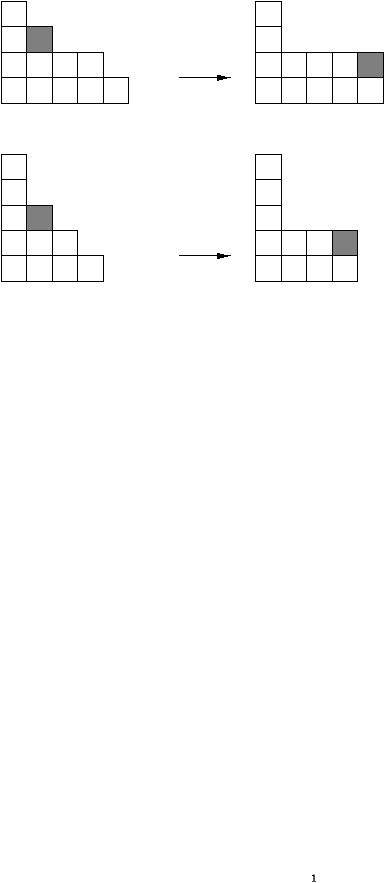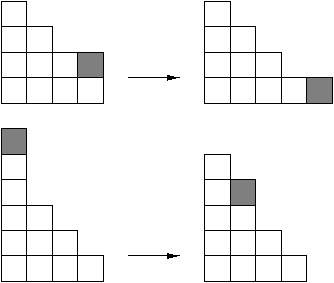(a)(b)

Figure 1: (a) Application of horizontal rule to (5,4,2,1) and (4,3,2,1,1); (b) application of vertical rule to (4,4,2,1) and (4,3,2,1,1,1).

In the SPM(k) model (Sand Pile Model), introduced by Goles and Kiwi , the initial configuration is made of one column of n grains, and the only available rule is the vertical rule.

### 1.2  Generating function

Let p(n,k) denote the number of partitions of n of width k. Then:
F(q,x)=1+
 å n,k³1
p(n,k)qnxk =xqF(q,x)+F(q,xq)
=
 ¥ Õ i=1
1
1-xqi
.

### 1.3  Example of bijection

There is a bijection between partitions with odd parts and partitions with distinct parts, as is reflected by the generating functions identity
 ¥ Õ i=1
1
1-q2i+1
=
 ¥ Õ i=1
1-q2i
1-qi
=
 ¥ Õ i=1
( 1+qi ) .

### 1.4  Order on partitions

Let µ=(µ12,...) and l=(l1,l2,...) be two partitions of n. We say that µ³l if and only if there exists a sequence of moves of n induced by the rules to go from µ to l. In the SPM(k) model, this order is equivalent to the dominance order LB(n)  on the conjugates: µ³l if and only if åi=1jµ'i³åi=1jl'i for all j³1. Brylawski  showed:
Theorem 1   Let n be an integer. The set of partitions of n with the previously defined order is a lattice, where the maximal element is (1,1,...,1), and the minimal element is (n). Moreover, the infimum and the supremum of two partitions can be respectively defined as follows:
1. inf(µ,l)=p such that p'j=min(åi=1jµ'i,åi=1jl'i) -åi=1jp'i for all j³1.
2. sup(µ,l)=a such that a'j=max(åi=1jµ'i,åi=1jl'i) -åi=1ja'i for all j³1.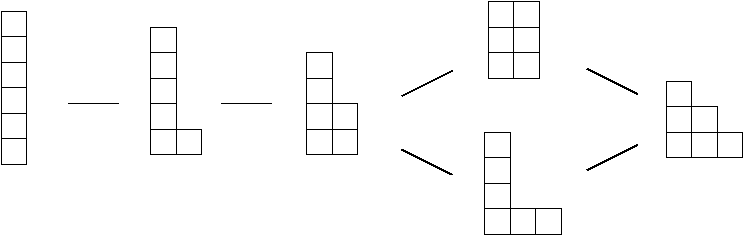Figure 2: LB(6)

In Figure 2, the maximal element (1,1,1,1,1,1) is on the left.

##### Length of a maximal chain
The length of a maximal chain is greater than 2n-3 , and smaller than 2(
 l+1 3
)+lj+1 , where l and j are defined by n=j+l(l+1)/2 and 0£ j£ l. For n=6, the two bounds are equal to 9, which shows that they both can be attained. The corresponding maximal chain is displayed in Figure 2.

## 2  IPM(k) Model

A more realistic generalization of SPM(k) model limits the lengths of the possible horizontal shifts of a grain.

### 2.1  Definition

In , the sand piles in IPM(k) are characterized in the following way:
Proposition 1   A sand pile in IPM(k) is a partition p=(p1,...,pl) of n such that
• for 1£ i£ l, 0£pi-pi+1£ k+1;
• for any i<j with pi-pi+1=k+1 and pj-pj+1=k+1, there exists z with i<z<j such that pz-pz+1< k.

### 2.2  Generating functions

#### 2.2.1  Area and height

Theorem 2   The generating function Sk(q,x) of IPM(k) sand piles, with q and x respectively counting area and height, satisfies
Sk(q,x)=1+
 å pÎIPM(k)
xl(p)q|p| =1+
 k å i=1
xqi
1-xqi
Sk(q,xqi) +xqk+1Sk(q,xqk).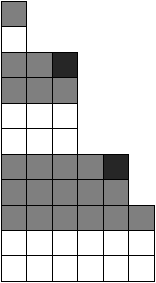Figure 3: Decomposition of a sand pile in IPM(1).

Proof. A sand pile in IPM(k) is either the empty partition, or a partition in IPM(k) where one duplicates i times the highest column and adds to it at least one part i (1£ i£ k), or a partition in IPM(k) where one duplicates k times the highest column and adds to it one part of length k+1. This decomposition yields the last expression for Sk(q,x) in the statement of the theorem, after noting that Sk(q,xqr) is the generating function obtained by duplicating r times the highest column in each sand pile.

Note the particular cases:
S1(q,x)=1+
 å n³1
xnqn(n+1)/2
 n Õ i=1
æ
ç
ç
è
q+
1
1-xqi
ö
÷
÷
ø
;     S¥(q,x)=
 n Õ i=1
æ
ç
ç
è
q+
1
1-xqi
ö
÷
÷
ø
.

#### 2.2.2  Area and width

Theorem 3   The generating function Sk(q,y) of IPM(k) sand piles, with q and y respectively counting area and width, satisfies:
Sk(q,y)= æ
ç
ç
è
1-(yq)k+1
1-yq
+ykqk-1 ö
÷
÷
ø
Sk(q,yq)+ykqk-1 ( Sk(q,yq)-Sk(q,yq2) ) .

In particular:
S 1(q,y)=1+
 å n³1
ynqn(n-1)/2
 n Õ i=1
1+q-qi-1
1-qi
.

#### 2.2.3  Height and width

Let pk(h,w) be the number of sand piles in IPM(k) of height h and width w and Pk,h(y) the generating function åw³0pk(h,w)yw.
Theorem 4   The generating function Pk,h(y) follows the recurrence:
Pk,0(y)=1;     Pk,1(y)=y
1-yk+1
1-y
;     Pk,2(y)=y
1-yk+1
1-y
1-yk+2
1-y
-y2(k+1);
Pk,h(y)= æ
ç
ç
è
1-yk+1
1-y
+yk ö
÷
÷
ø
Pk,h-1(y)-ykPk,h-2(y)     for h³3.

Now, let Pk(x,y) be the width (variable x) and the height (variable y) generating function åh³0Pk,h(y)xh. From the previous recurrence one gets:
Theorem 5   The generating function Pk(x,y) is given by:
Pk(x,y)=
1-x(yk+1-yk+1)+x2yk(1-y)
1-x æ
ç
ç
è
1-yk+1
1-y
+yk(1-x) ö
÷
÷
ø
.

Let pk(n) be the number of sand piles in IPM(k) of half perimeter (width + height) n, and Pk(x)=ån³0pk(n)xn be its generating function. As Pk(x)=Pk(x,x), its expression is:
Pk(x)=
(1-x)2(1-xk+1+xk+2)
1-2x-3xk+2+xk+3+xk+1
.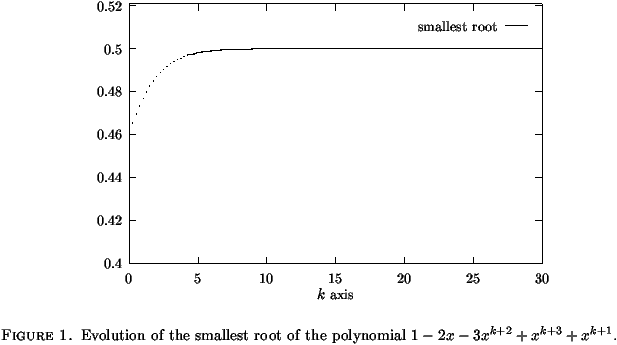When k grows, the quantity pk(n), asymptotically equal for large n to ck/rkn with ckÎR and rk the smallest root of the denominator 1-2x-3xk+2+xk+3+xk+1, gets closer to 2n, the number of partitions of semi-perimeter n.

### 2.3  Asymptotics

Define
pk=[qn]
 Õ i³1
1-qki
1-qi
,     Bk=(
k-1
6k
)1/2,    and     Ck=
1
2
æ
ç
ç
è
k-1
6k3
ö
÷
÷
ø
1/4.
Then pk(n)=Ckn-3/4exp(Bkn1/2)O(1+n-1/4). If Ik(n) is the number of partitions of n in IPM(k), then pk+1(n)£ Ik(n)£ pk+2(n).

## 3  The Model L(q)

The model L(q) generalizes the SPM(k) by restricting its vertical rule, instead of the horizontal rule as IPM(k). Namely, the difference between the two consecutive columns involved must be greater then q.

### 3.1  Definition

In , the sand piles in L(q) are characterized in the following way:
Proposition 2   A sand pile in L(q) is a partition p=(p1,...,pl) of n such that
• for 1£p1, p'i-p'i+1³q-1;
• for any i<j with p'i-p'i+1=q-1 and p'j-p'j+1=q-1, there exists z with i<z<j such that pz-pz+1>q.

Let Lq(q,x)= 1+åpÎ L(q)xl(p)q|pi| the generating function of sand piles in L(q) according to their height and area.
Lemma 1   Lq(q,x) satisfies the q-equation:
Lq(q,x)=
1-(xq)q-1
1-xq
+ æ
ç
ç
è
(xq)q-1
1-xq
+xq-1qq ö
÷
÷
ø
Lq(q,xq).

Theorem 6   Lq(q,x) is given by:
L q(q,x)=
 å n³0
xq nqq n(n+1)/2
1-(xqn+1)q
1-xqn+1
 n Õ i=1
æ
ç
ç
è
q+
1
1-xqi
ö
÷
÷
ø
.

Bounds can be obtained for all q for the number ln,q of partitions in L(n,q).

## 4  Frobenius Model

Another generalization consists in allowing the grains to move both to the left and to the right. In , Corteel defines such a model, called the Frobenius sand pile, in the following way:
Definition 1   Let l be an integer. A Frobenius sand pile is a pair consisting of a pivot indice p(a)£ l and a sequence of integers (a1,a2,...,al) such that
a1£ a2£ ...£ ap(a) ³ ap(a)+1³...³ al.

### 4.1  Order on Frobenius sand piles

Definition 2   Let a=(p(a),(a1,a2,...,al)) and b=(p(b),(b1,b2,...,bl)) be two Frobenius sand piles. Then a³Fb if and only if, for all i,j³0,
 p(a)+j å l=p(a)-i
al³
 p(b)+j å l=p(b)-i
bl.

Proposition 3   Let LF(n) be the set of Frobenius partitions ordered by ³F. Then LB(n) is a suborder of LF(n).

##### Length of a maximal chain
For n³3, the length of a maximal chain is greater than 2n-4, and smaller than 2(
 l+1 3
)+lj+1, where l and j are defined by n=j+l(l+1)/2 and 0£ j£ l.
Definition 3   Let a=(p(a),(a1,a2,...,al)) be a sand pile. a<, a>, a£, and a³ are defined by
a<
 = ( ap(a)-1,ap(a)-1,...,a1 ) ;
a>
 = ( ap(a)+1,ap(a)+2,...,al ) ;

a£
 = ( ap(a),ap(a)-1,...,a1 ) ;
a³
 = ( ap(a),ap(a)+1,...,al ) .

If we constrain horizontal shifts to be smaller than k, we can create an increasing sequence of orders IFPM(k) with the relations of order ³k. The Frobenius sand piles of IFPM(k) are characterized by:
Proposition 4   Let a=(p(a),(a1,a2,...,al)) be a sand pile. This sand pile belongs to IFPM(k) if and only if both of a< and a>, and at least one of a£ and a³ belong to IPM(k).

### 4.2  Generating functions

The only available generating function is the series of F-partitions given by
1+
 å k³1
qk
 k Õ i=1
1
 ( 1-qi ) 2
.
For IFPM(k), we must so far satisfy ourselves with the bound Fk(n)£ |IFPM(n,k)| £ Fk+1(n) for
Fk(n)=[qn] æ
ç
ç
è
1+
 å j³1
qj
 j Õ i=1
1-q(k+1)i
 ( 1-qi ) 2
ö
÷
÷
ø
.

## 5  Conclusion and Open Questions

We have studied different sand pile models related to integer partitions, and in particular we have computed generating functions and asymptotic bounds. A question of interest would consist in getting exact asymptotics instead of asymptotic bounds only. One could start with the area generating function in the SPM case, given by
 å n³0
xnqn(n+1)/2
 n Õ i=1
æ
ç
ç
è
q+
1
1-qi
ö
÷
÷
ø
.

## References


Brylawski (Thomas). -- The lattice of integer partitions. Discrete Mathematics, vol. 6, 1973, pp. 201--219.


Corteel (Sylvie). -- Problèmes énumératifs issus de l'Informatique, de la Physique et de la Combinatoire. -- Thèse, Université Paris-Sud, 2000.


Goles (Eric) and Kiwi (Marcos A.). -- Games on line graphs and sand piles. Theoretical Computer Science, vol. 115, n°2, 1993, pp. 321--349.


Phan (Ha Duong). -- Structures ordonnées et piles de sable. -- Thèse, Université Paris 7, 1998.

This document was translated from LATEX by HEVEA.